﻿ 基于粗糙集和神经网络的柴油机转子振动故障模式识别 Fault Pattern Recognition of Diesel Rotor Vibration Based on Rough Set and Neural Network

Computer Science and Application
Vol.08 No.03(2018), Article ID:24291,9 pages
10.12677/CSA.2018.83043

Fault Pattern Recognition of Diesel Rotor Vibration Based on Rough Set and Neural Network

Liang Sang1, Dong Li2, Tao Sun3, Liantao Li2

1The Unit of 91245 Army, Huludao Liaoning

2The Unit of 91899 Army, Huludao Liaoning

3Aviation Fundamental Academy Naval Aviation University, Yantai Shangdong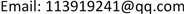Received: Mar. 9th, 2018; accepted: Mar. 22nd, 2018; published: Mar. 30th, 2018ABSTRACT

A method of diesel rotor vibration fault pattern recognition based on rough set and neural network was proposed. Aiming at huge of collected data and computation, representative point set determination method was proposed, representative point set demonstrated original point, an abnormal point was ticked off. Wavelet packet of representative point set was calculated, energy feature of vibration signal was extracted. On the basis of different data discrete methods, the discrete method was improved; a combined discrete method was determined by data construction. Finally, faults were diagnosed by BP network and simplified rule. The fault diagnosis method was applied to diesel, which is feasible.

Keywords:Rough Set, Diesel Rotor Vibration, Fault Diagnosis, Representative Point, Neural Network

191245部队，辽宁 葫芦岛

291899部队，辽宁 葫芦岛

3海军航空大学，航空基础学院，山东 烟台1. 引言

2. 故障模式识别

3. 数据代表点集

${V}_{\epsilon }\left({x}_{i}\right)$ 为样本 ${x}_{i}$ 的e-邻域，它是以 ${x}_{i}$ 为中心，以e为半径的超球，满足条件“任取 $x\in {V}_{\epsilon }\left({x}_{i}\right)$$‖x-{x}_{i}‖\le \epsilon$ ”。而 ${x}_{i}$ 处的密度表示表示位于 ${V}_{\epsilon }\left({x}_{i}\right)$ 中训练样本的个数，即 ${N}_{\epsilon }\left({x}_{i},X\right)$ ，其中 $X=\left\{{x}_{i}\right\}$ 是样本集。进一步，如果P满足：

1) $P\subseteq X$ ;

2) $\forall p\in P,{N}_{\epsilon }\left(p,X\right)\le q$ ;

3) $\forall x\in X,\text{\hspace{0.17em}}\exists p\in P,\text{\hspace{0.17em}}\text{satisfy}\text{\hspace{0.17em}}x\in {V}_{\epsilon }\left(p\right)$

$\begin{array}{l}\text{Set}\text{\hspace{0.17em}}{X}^{un}=X,\text{\hspace{0.17em}}P=\phi \\ \text{While}\text{\hspace{0.17em}}{X}^{un}\ne \Phi ,\text{do}\\ \text{\hspace{0.17em}}\text{\hspace{0.17em}}\text{\hspace{0.17em}}\text{\hspace{0.17em}}\text{\hspace{0.17em}}\text{\hspace{0.17em}}\text{\hspace{0.17em}}\text{\hspace{0.17em}}\text{Arbitrarily}\text{\hspace{0.17em}}\text{select}\text{\hspace{0.17em}}a\text{\hspace{0.17em}}x\in {X}^{un}\\ \text{\hspace{0.17em}}\text{\hspace{0.17em}}\text{\hspace{0.17em}}\text{\hspace{0.17em}}\text{\hspace{0.17em}}\text{\hspace{0.17em}}\text{\hspace{0.17em}}\text{\hspace{0.17em}}\text{If}\text{\hspace{0.17em}}{N}_{\epsilon }\left(x,P\right)\le q,\text{\hspace{0.17em}}\text{set}\\ \text{\hspace{0.17em}}\text{\hspace{0.17em}}\text{\hspace{0.17em}}\text{\hspace{0.17em}}\text{\hspace{0.17em}}\text{\hspace{0.17em}}\text{\hspace{0.17em}}\text{\hspace{0.17em}}-P=P+\left\{x\right\}\\ \text{\hspace{0.17em}}\text{\hspace{0.17em}}\text{\hspace{0.17em}}\text{\hspace{0.17em}}\text{\hspace{0.17em}}\text{\hspace{0.17em}}\text{\hspace{0.17em}}\text{\hspace{0.17em}}-{X}^{un}={X}^{un}-\left\{x\right\}\\ \text{\hspace{0.17em}}\text{\hspace{0.17em}}\text{\hspace{0.17em}}\text{\hspace{0.17em}}\text{\hspace{0.17em}}\text{\hspace{0.17em}}\text{\hspace{0.17em}}\text{\hspace{0.17em}}\text{End}\text{\hspace{0.17em}}\left\{\text{if}\right\}\\ \text{End}\text{\hspace{0.17em}}\left\{\text{while}\right\}\end{array}$ (1)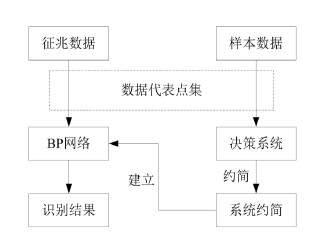Figure 1. Fault pattern recognition based on rough set and neural network

4. 数据特征提取

1) 选取适当的小波包函数，选取sym7小波；

2) 确定分解层数L，本文 $L\ge 5$

3) 根据先验知识和每个序列的起始频率，计算出位于第L层的某几个频段内的频率成分；

4) 对数据进行逐层小波包分解。分解第l层时得到位于不同频段的 ${2}^{l-1}$ 组序列。每组序列分别由低通滤波结果 ${W}_{2n}^{l}$ 和高通滤波结果 ${W}_{2n+1}^{l}$ 组成。

5) 利用回复公式重构信号。

$E\left(j,k\right)=\frac{1}{N}\sum _{i=1}^{M}{\left[{d}_{l}\left(j,k\right)\right]}^{2},\text{\hspace{0.17em}}\left(l=1,2,\cdots ,N\right)$ (2)

$E\left(0,0\right)=\sum _{i=1}^{M}{E}_{i}\left(j,k\right)$ (3)

$e={\left\{{E}_{1},{E}_{2},\cdots ,{E}_{M}\right\}}^{T}/E\left(0,0\right)$ (4)

5. 粗糙集与数据离散化方法

$S=\left(U,A,V,f\right)$ 是一个知识表达系统，其中U表示对象的非空有限集合，称为论域； $A=C\cup D$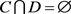，C称为条件属性集，D称为决策属性集； $V=\underset{a\in A}{\cup }{V}_{a}$ 是属性a的值域；f表示 $U×A\to V$ 是一个信息函数，它为每个对象的每个属性赋予一个信息值，即： $\forall a\in A,x\in U,f\left(x,a\right)\in V$ 。具有条件属性和决策属性的知识表达系统称为决策表。两个属性集C与D之间的依赖程度 ${\gamma }_{C}\left(D\right)$ 定义为

${\gamma }_{C}\left(D\right)=\frac{|po{s}_{C}\left(D\right)|}{|U|}$ (5)

${\sigma }_{CD}\left(a\right)={\gamma }_{C}\left(D\right)-{\gamma }_{C-\left\{a\right\}}\left(D\right)$ (6)

6. 基于粗糙集和神经网络的故障模式识别

$\begin{array}{c}d\left(0,0\right)=d\left(5,0\right)+d\left(5,1\right)+d\left(5,2\right)+d\left(5,3\right)+d\left(5,4\right)+d\left(5,5\right)\\ \text{\hspace{0.17em}}\text{\hspace{0.17em}}+d\left(4,3\right)+d\left(4,4\right)+d\left(4,5\right)+d\left(3,3\right)+d\left(1,1\right)\end{array}$ (7)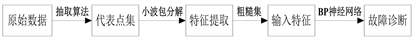Figure 2. System of fault pattern recognition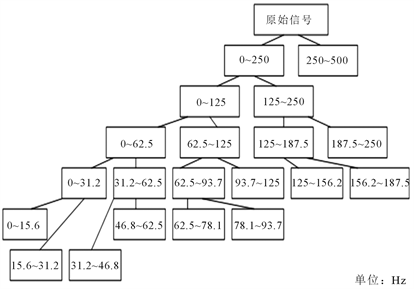Figure 3. Improved decomposition tree

1) 本文故障模式识别与其他方法对比Table 1. The corresponding frequency bands and energy characteristics of wavelet packet decomposition coefficients of misalignment faultTable 4. Comparison of pattern recognition and other methods

2) 代表点集与原始数据特征提取模式识别对比

3) 未经特征提取与特征提取后模式识别对比

7. 总结

1) 本文设计的故障识别系统达到了很高的精度。选取了原始数据代表点集，极大的减少了计算量，同时代表点集又可以表示原始数据全部信息，可以达到很高的诊断精度。

2) 代表点集经过特征提取和粗糙集处理后，能够得到最简化的最直接的与故障相关的特征，诊断精度大为提高。并且本文故障识别系统与SVM多分类与k-means识别方法相比，计算用时与精度都有所提高。

3) 以最简化的诊断规则作为输入，带入到BP神经网络中，不能涵盖所有故障样本，此时增加条件属性数量，带入到神经网络输入中，可以提高识别断精度。Table 5. Comparison of representative point and original dataTable 6. Comparison of representative point direct input and feature extraction

Fault Pattern Recognition of Diesel Rotor Vibration Based on Rough Set and Neural Network[J]. 计算机科学与应用, 2018, 08(03): 389-397. https://doi.org/10.12677/CSA.2018.83043

1. 1. 徐启华, 师军, 耿帅. 应用快速多分类SVM的航空发动机故障诊断方法[J]. 推进技术, 2015, 33(6): 961-967.

2. 2. 栗茂林, 王孙安, 梁霖. 利用非线性流形学习的轴承早期故障特征方法[J]. 西安交通大学学报, 2008, 44(5): 45-50.

3. 3. 黄向华, 丁毅. 基于几何模式识别的发动机传感器故障诊断[J]. 航空学报, 2006, 27(6): 1018-1022.

4. 4. 胡金海, 谢寿生, 侯胜利, 等. 粗糙核主元分析方法及其在故障特征提取中的应用[J]. 振动与冲击, 2015, 27(3): 50-54.

5. 5. 赵凯. 基于粗糙集的转子振动信号挖掘技术研究[D]: [硕士学位论文]. 烟台: 海军航空工程学院, 2010.

6. 6. 胡雷, 胡鸾庆, 秦国军, 等. 涡轮泵状态监控及传感器故障识别的新异类检测方法[J]. 国防科技大学学报, 2010, 32(2): 119-123.

7. 7. 曾庆生, 王湘江. 基于小波能量谱和粗糙集的离心式压缩机振动故障诊断[J]. 中南大学学报(自然科学版), 2009, 40(3): 706-710.

8. 8. 胡金海, 谢寿生, 胡剑锋, 等. 基于粗糙集理论的航空发动机性能综合评判[J]. 系统工程与电子技术, 2006, 28(5): 704-707.

9. 9. 蒋延军, 倪远平. 基于粗糙集与支持向量机的变压器故障诊断法[J]. 高电压技术, 2008, 34(8): 1755-1760.

10. 10. 谭旭, 唐云岚, 张少丁, 等. 杂合数据的粗糙集属性约简方法[J]. 国防科技大学学报, 2008, 30(6): 83-88.

11. 11. 黄文涛, 王伟杰, 赵学增, 等. 从不完备数据中获取诊断规则的粗糙集方法[J]. 电力系统自动化, 2005, 29(14): 49-54.